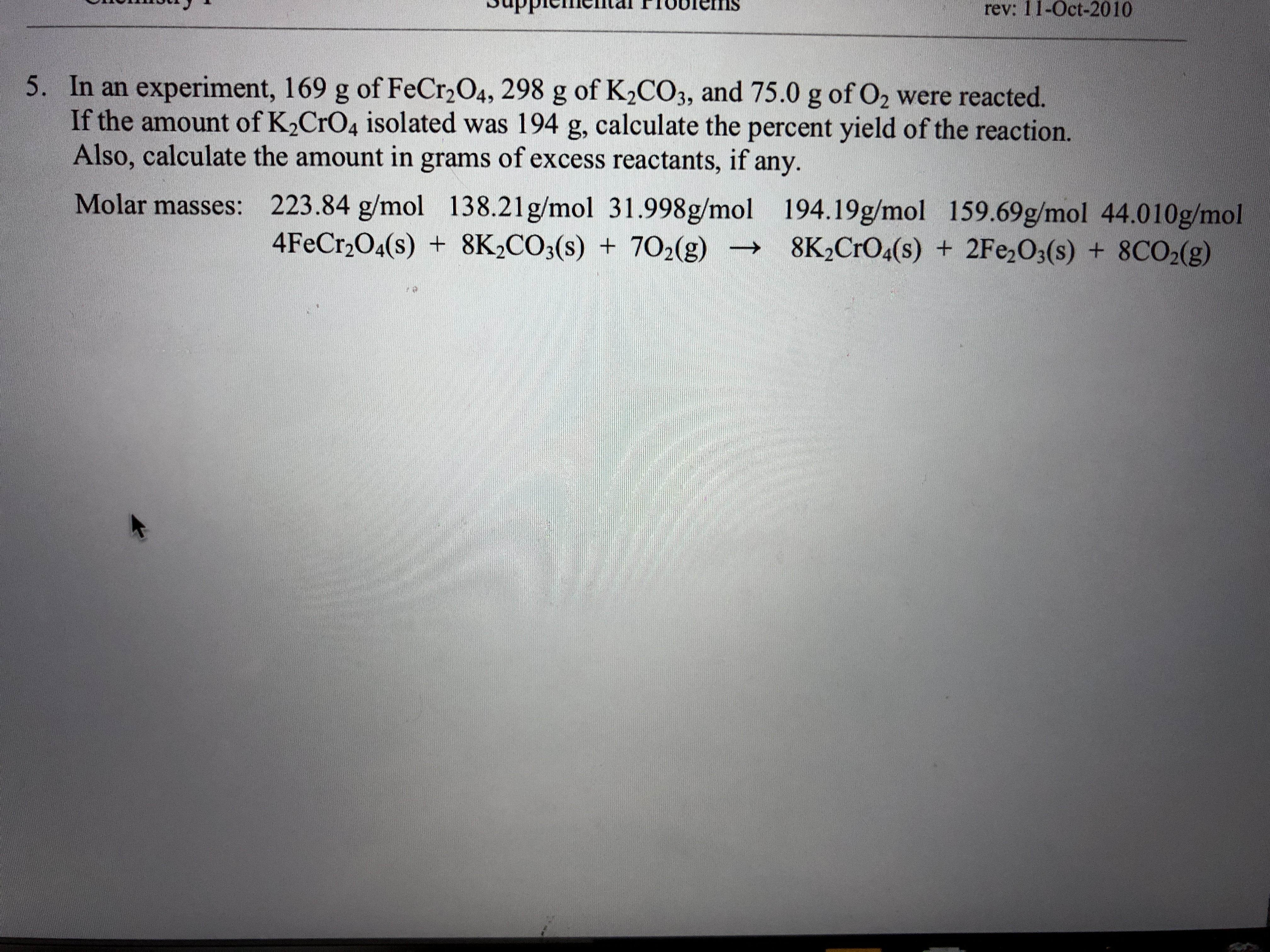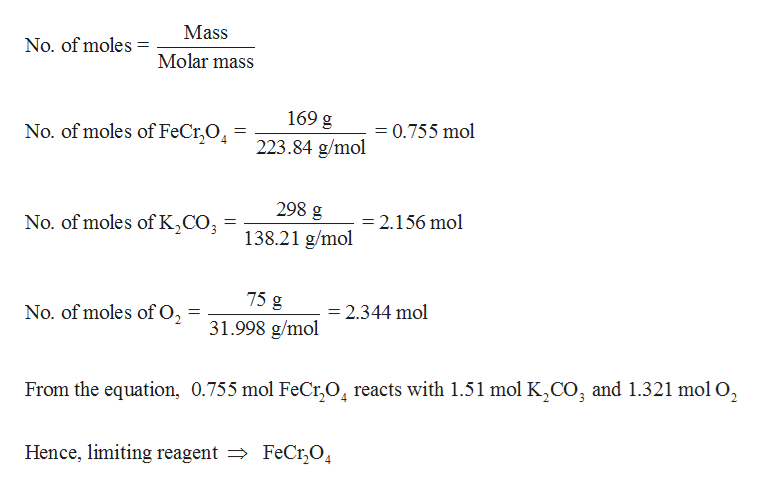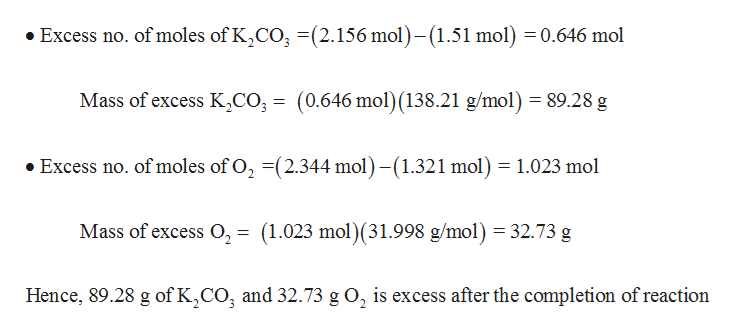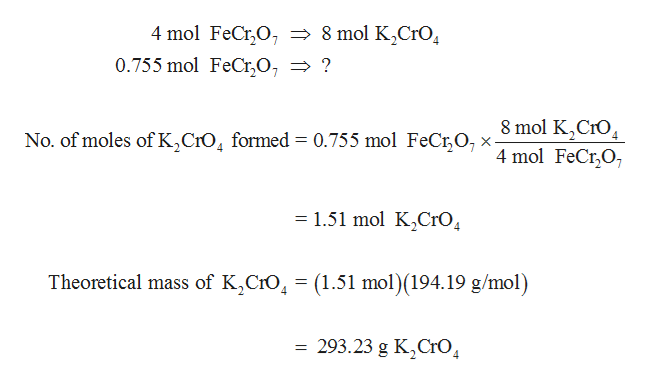# rev: 11-Oct-20105. In an experiment, 169 g of FeCr2 O4, 298 g of K2CO3, and 75.0 g of O2 were reacted.If the amount of K2CrO4 isolated was 194 g, calculate the percent yield of the reaction.Also, calculate the amount in grams of excess reactants, if any.223.84 g/mol 138.21g/mol 31.998g/mol 194.19g/mol 159.69g/mol 44.010g/mol4FeCr2O4(s) 8K2CO3(s)Molar masses:+702(g)8K2CrO4(s) + 2Fe O3(s)+ 8CO2(g)

Question
8 views

How do you solve this?help_outlineImage Transcriptioncloserev: 11-Oct-2010 5. In an experiment, 169 g of FeCr2 O4, 298 g of K2CO3, and 75.0 g of O2 were reacted. If the amount of K2CrO4 isolated was 194 g, calculate the percent yield of the reaction. Also, calculate the amount in grams of excess reactants, if any. 223.84 g/mol 138.21g/mol 31.998g/mol 194.19g/mol 159.69g/mol 44.010g/mol 4FeCr2O4(s) 8K2CO3(s) Molar masses: +702(g) 8K2CrO4(s) + 2Fe O3(s) + 8CO2(g) fullscreen
check_circle

Step 1

Determine the limiting reagent:help_outlineImage TranscriptioncloseMass No. of moles Molar mass 169 g 0.755 mol No. of moles of FeCr,O - 223.84 g/mol 298 g 138.21 g/mol = 2.156 mol No. of moles of K2CO 75 g =2.344 mol No. of moles of O2 31.998 g/mol From the equation, 0.755 mol FeCr04 reacts with 1.51 mol K2CO, and 1.321 mol O2 FeCr,04 Hence, limiting reagent fullscreen
Step 2

Mass of excess reactants (K2CO3 and O2) is determined as follows,help_outlineImage TranscriptioncloseExcess no. of moles of K2CO (2.156 mol)- (1.51 mol) 0.646 mol Mass of excess K,co, = (0.646 mol) (138.21 g/mol) = 89.28 g Excess no. of moles of O, (2.344 mol)-(1.321 mol) 1.023 mol Mass of excess O2 = (1.023 mol) (31.998 g/mol) 32.73 g Hence, 89.28 g of K,CO, and 32.73 g O2 is excess after the completion of reaction fullscreen
Step 3

From the equation, it is clear that 4 mol of FeCr2O7 reacts to give 8 mol of K2CrO4. Hence, the theoretical ...help_outlineImage Transcriptionclose4 mol FeCr,0, - 8 mol K2C1O 0.755 mol FeCr,O7 > ? 8 mol K CrO 4 mol FeCr,O No. of moles of K,CrO4 formed = 0.755 mol FeCrO, x- 1.51 mol K2CrO 4 Theoretical mass of K,CrO4 (1.51 mol)(194.19 g/mol) 293.23 g K CrO fullscreen

### Want to see the full answer?

See Solution

#### Want to see this answer and more?

Solutions are written by subject experts who are available 24/7. Questions are typically answered within 1 hour.*

See Solution
*Response times may vary by subject and question.
Tagged in

### General Chemistry